Test Description

## 15 Questions MCQ Test Mental Mathematics | Test: Understanding Quadrilaterals- 2

Test: Understanding Quadrilaterals- 2 for Class 7 2023 is part of Mental Mathematics preparation. The Test: Understanding Quadrilaterals- 2 questions and answers have been prepared according to the Class 7 exam syllabus.The Test: Understanding Quadrilaterals- 2 MCQs are made for Class 7 2023 Exam. Find important definitions, questions, notes, meanings, examples, exercises, MCQs and online tests for Test: Understanding Quadrilaterals- 2 below.
Solutions of Test: Understanding Quadrilaterals- 2 questions in English are available as part of our Mental Mathematics for Class 7 & Test: Understanding Quadrilaterals- 2 solutions in Hindi for Mental Mathematics course. Download more important topics, notes, lectures and mock test series for Class 7 Exam by signing up for free. Attempt Test: Understanding Quadrilaterals- 2 | 15 questions in 30 minutes | Mock test for Class 7 preparation | Free important questions MCQ to study Mental Mathematics for Class 7 Exam | Download free PDF with solutions
 1 Crore+ students have signed up on EduRev. Have you?
Test: Understanding Quadrilaterals- 2 - Question 1

### Complete the following : While constructing a quadrilateral with three sides and two diagonals_______triangles are made on the same base.

Detailed Solution for Test: Understanding Quadrilaterals- 2 - Question 1

Two

Test: Understanding Quadrilaterals- 2 - Question 2

### Complete the following: A quadrilateral can be constructed uniquely if 4 sides and one _________ is known.

Test: Understanding Quadrilaterals- 2 - Question 3

### The side of the square is 16 cm. What is the length of the diagonal?

Detailed Solution for Test: Understanding Quadrilaterals- 2 - Question 3

16√2 cm

Test: Understanding Quadrilaterals- 2 - Question 4

ABCD is a square with sides 2.4 cm. What is the perimeter of the square?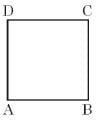Detailed Solution for Test: Understanding Quadrilaterals- 2 - Question 4

9.6 cm

Test: Understanding Quadrilaterals- 2 - Question 5

What is the length of the rectangle if its breadth is 10 cm and the diagonal is 26 cm?

Detailed Solution for Test: Understanding Quadrilaterals- 2 - Question 5

24 cm

Test: Understanding Quadrilaterals- 2 - Question 6

Which figure is this?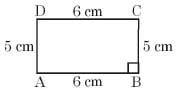Detailed Solution for Test: Understanding Quadrilaterals- 2 - Question 6

rectangle

Test: Understanding Quadrilaterals- 2 - Question 7

PQRS is a rhombus with PQ = 10 cm. If OQ = 6 cm then what is the length of the diagonal PR?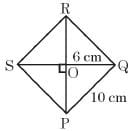Detailed Solution for Test: Understanding Quadrilaterals- 2 - Question 7

16 cm

Test: Understanding Quadrilaterals- 2 - Question 8

PQRS is a rhombus. If PO = 4 cm and OQ = 3 cm then what is PR + SQ?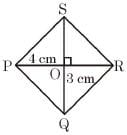Test: Understanding Quadrilaterals- 2 - Question 9

Complete the following:
The diagonals of a parallelogram _________ each other.

Test: Understanding Quadrilaterals- 2 - Question 10

In a parallelogram ABCD the point of intersection of both diagonals AC and BD is O.
If AC = 16 cm and BD = 12 cm then what is OA and OD?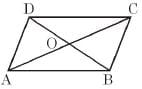Test: Understanding Quadrilaterals- 2 - Question 11

Complete the following:
The opposite angles of a parallelogram are _________.

Test: Understanding Quadrilaterals- 2 - Question 12

In a parallelogram ∠F : ∠G = 2 : 3 . What is the degree measure of ∠F and ∠G?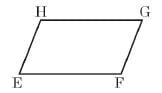Test: Understanding Quadrilaterals- 2 - Question 13

ABCD is a parallelogram. If ∠C 80° then w hat is ∠B + ∠D?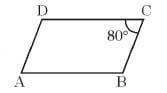Test: Understanding Quadrilaterals- 2 - Question 14

he ratio of two adjacent sides of a parallelogram is 5:4. Its perimeter is 18 cm then, what is the length of the adjacent sides?

Detailed Solution for Test: Understanding Quadrilaterals- 2 - Question 14

5 cm, 4 cm

Test: Understanding Quadrilaterals- 2 - Question 15

Complete the following:
The opposite sides of a parallelogram are _____.

## Mental Mathematics

49 videos|30 tests
 Use Code STAYHOME200 and get INR 200 additional OFF Use Coupon Code